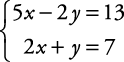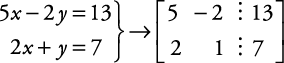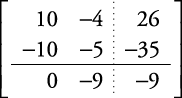## Linear Equations: Solutions Using Matrices with Two Variables

A matrix (plural, matrices) is a rectangular array of numbers or variables. A matrix can be used to represent a system of equations in standard form by writing only the coefficients of the variables and the constants in the equations.

##### Example 1

Represent this system as a matrix.In the preceding matrix, the dashed line separates the coefficients of the variables from the constants in each equation.

Through the use of row multiplication and row additions, the goal is to transform the preceding matrix into the following form.The matrix method is the same as the elimination method but more organized.

##### Example 2

Solve this system by using matrices.Multiply 2 times row 1 and –5 times row 2; then add:This matrix now represents the systemTherefore,  y = 1

Now, substitute 1 for y in the other equation and solve for x.Check the solution.The solution is x = 3, y = 1.

Matrices are a more time‐consuming method of solving systems of linear equations than either the elimination or substitution methods. They only become a time‐saving method when solving multiple equations in multiple variables that are repeatedly equated to different sets of constants. Don't worry; you won't have to do those this year. Still, you should know that they are an alternative method of solving linear equation systems.